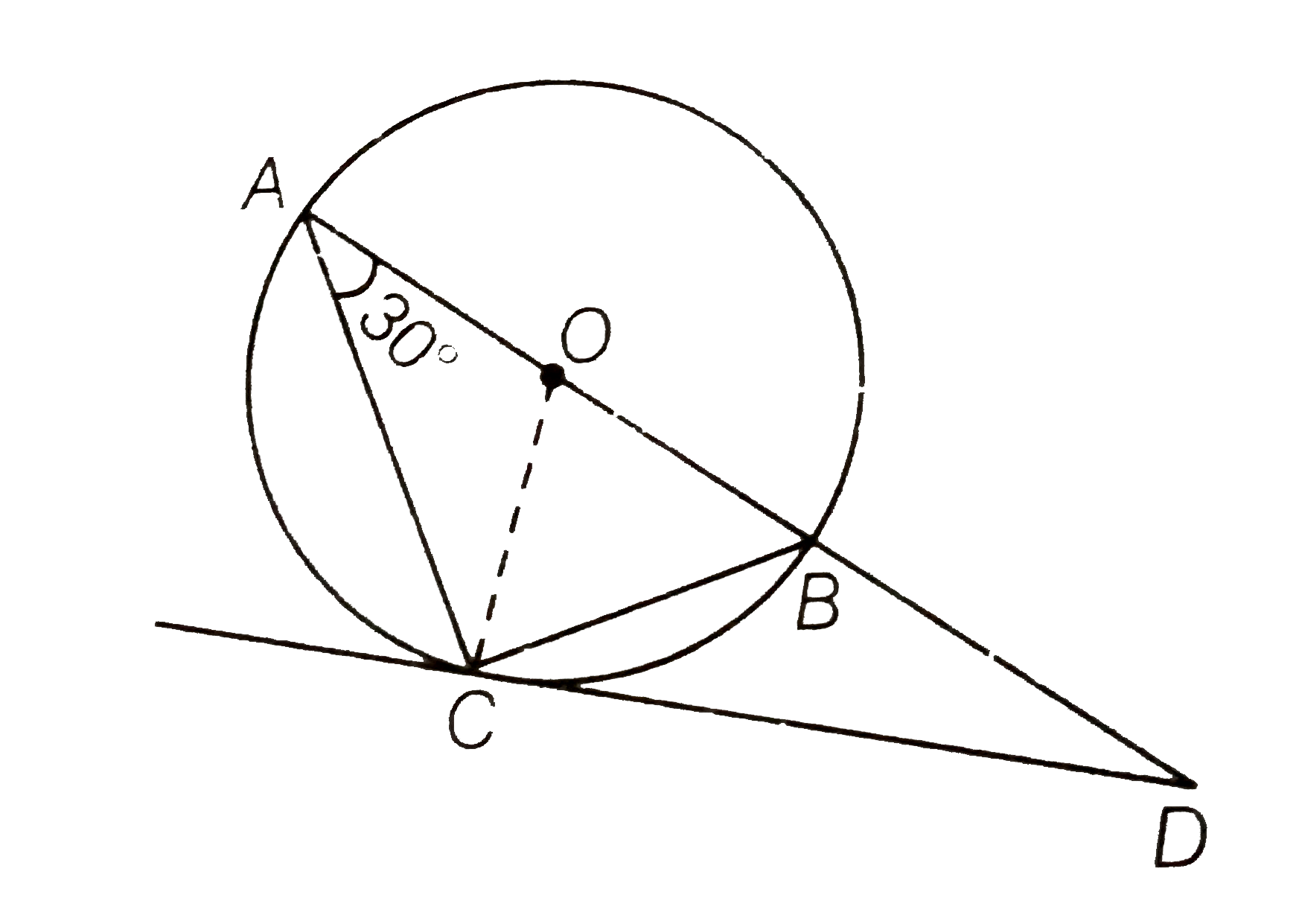Doubtnut App - Get 50 Lakh+ video solutions better than GoogleInstall Now

# AB is a diameter of a circle and AC is its chord such that ∠BAC=30∘. If the tengent at C intersects AB extended at D, then BC=BD.

Text Solution
Verified by Experts

## To Prove, BC=BDJoin BC and OC. Given, ∠BAC=30∘ ⇒∠BCD=30∘ [angle between tangent and chord is equal to angle made by chord in the alternate segment] ∴∠ACD=∠ACO+∠OCD=30∘+90∘=120∘ [∴OC⊥CDandOA=OC=radius⇒∠OAC=∠OCA=30∘] In ΔACD, ∠CAD+∠ACD+∠ADC=180∘ [since, sum of all interior angles of a triangle is 180∘] ⇒30∘+120∘+∠ADC=180∘ ⇒∠ADC=180∘−(30∘+120∘)=30∘ Now in ΔBCD ∠BCD=∠BDC=30∘ ⇒BC=BD [since, sides opposite to equal angles are equal]